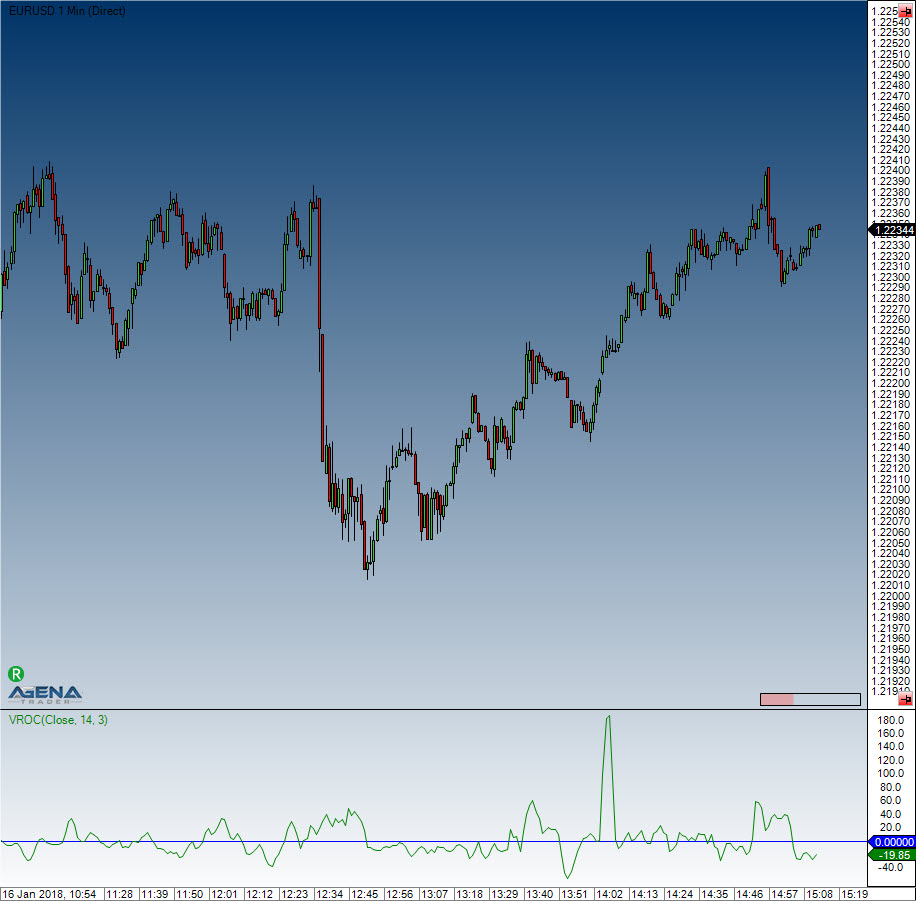Comment on page

# Volume Rate of Change (VROC)

#### Description

The Volume Rate of Change (VROC) is almost exactly the same as the ROC indicator, with the exception that instead of price data, volume data (VOL) is used. A smoothing component is also applied.

#### Usage

VROC(int period, int smooth)
VROC(IDataSeries inSeries, int period, int smooth)
VROC(int period, int smooth)[int barsAgo]
VROC(IDataSeries inSeries, int period, int smooth)[int barsAgo]

#### Return value

double
When using this method with an index (e.g. VROC(14,3)[int barsAgo] ), the value of the indicator will be issued for the referenced bar.

#### Parameters

inSeries Input data series for the indicator
period Number of bars included in the calculations
smooth Number of Bars included in the calculation for the smoothing

#### VisualizationVolume Rate of Change (VROC)

#### Example

//Output of the current value for the Volume ROC
Print("The current value for the Volume ROC is: " + VROC(14, 3));